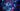# Quick Sort: Powerful and Elegant

June 10, 2021

·

·The other sorts we have covered each had some deficiency in terms of speed, space, or complexity. The sort that we cover now, the quick sort, achieves a perfect balance of all three metrics.

In the merge sort, we employed a recursive loop that involved splitting an array into its smallest sub-components and sorting each of those on the way up. With quick sort, we instead sort the top level array and then recurse through smaller components.

This method makes use of two facilities, `partition` and `sort`, in addition to the `exchange` function that we used in previous posts.

Let us start with the `partition` method.

``````template<typename T>
int partition(vector<T>& list, int low, int high)
{
int left = low;
int right = high + 1;
T pivot = list[low]; // arbitrarily pick low as pivot value

while (true) // loop until left and right meet
{
while (list[++left] < pivot) // update left until it hits out of place
{
if (left == high)
{
break;
}
}

while (pivot < list[--right]) // update right until it hits out of place
{
if (right == low)
{
break;
}
}

if (left >= right) // break when pointers meet
{
break;
}

exchange(list, left, right); // put lesser and greater on proper sides
}

exchange(list, low, right); // move pivot value into pivot position
return right;
};``````

This method starts by picking a `pivot` value. We arbitrarily set `pivot` to `low`, but we could also pick a random number between `low` and `high`. We also initialize `left` and `right` counters to point to the left and right ends of the relevant list range. We then loop indefinitely until `left` and `right` meet.

Along the way, we look for elements that are greater than `pivot` on the `left` side and elements that are less than `pivot` on the `right` side. Such elements are out of order with respect to `pivot`, and so we swap their places. When `left` and `right` meet, we put the `pivot` value at their center and return its index.

The `partition` method puts the list in a state where `pivot` is at the center of the relevant range, everything before `pivot` is less than `pivot`, and everything after `pivot` is greater than `pivot`.

By repeating this process on smaller and smaller sub-lists, the entire list is sorted. The `sort` method implements this recursive pattern.

``````template<typename T>
void sort(vector<T>& list, int low, int high)
{
if (high <= low) // base case
{
return;
}

const int mid = partition(list, low, high);
sort(list, low, mid - 1);
sort(list, mid + 1, high);
}``````

In this method, we call `partition` to find our pivot (sorting along the way), and then `sort` ranges to the left and right of the pivot. When `high` is less than or equal to `low`, the range has a size of 1 and the recursion ends.

To initiate this recursion, we call `sort` on the full range of the list, giving shape to our `quick_sort` method.

``````template<typename T>
void quick_sort(vector<T>& list)
{
sort(list, 0, list.size() - 1);
}``````

The quick sort is a powerful and elegant algorithm. In terms of speed, quick sort achieves optimal (linearithmic) complexity on average.

To avoid worst case performance, it is a good idea to shuffle the list prior to sorting.

In terms of space, the quick sort has a leg up on the merge sort (which requires an auxiliary list of n size) because it uses constant space in the form of a few counters. And in terms of complexity, the quick sort is fairly straightforward to understand and implement relative to its peers. For all of these reasons, for general applications, the quick sort is a safe first-choice.